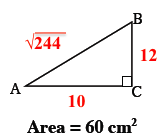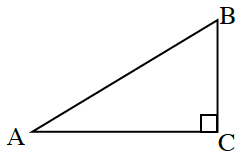### Home > CCA2 > Chapter 1 > Lesson 1.2.4 > Problem1-114

1-114.

The right triangle shown at right has a height of ( $m\overline{BC}$ ) of $12$ cm, and its area is $60$ square cm. Find $m∠B$ and the length of the hypotenuse.

$\text{Use }A=\frac{1}{2}bh\text{ and solve for }b.$

Use the answer from the previous hint to find the length of $\overline{AB}$.
Try using the Pythagorean Theorem.

$\text{Remember that tan}B=\frac{\text{opp}}{\text{adj}}.$

$m\measuredangle B\approx39.8^\circ\ \ \ \ \sqrt{244}\approx15.62$